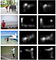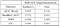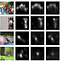# Image Saliency Prediction using Saliency GAN (SalGAN)

In my previous article I worte about how to predict saliency maps using convolutional neural networks (CNNs). This article will explore the GAN based architecture to predict saliency map proposed by Junting Pan et al. in their paper “SalGAN: Visual Saliency Prediction with Generative Adversarial Networks”.

“The function of wisdom is to discriminate between good and evil.” — Marcus Tullius Cicero

The GAN overview —

Generative adversarial networks (GANs) are generally used to synthesize images which have realistic data distribution. A conventional GAN model consists of two competing networks namely, a generator and a discriminator. The jobs of these networks are exactly as their name suggests. The generator produces samples whose data distribution is the same as that of the training set. The discriminator differentiates between the sample synthesized by the generator and the real sample drawn from the training set. The training of the GAN models proceeds by training the discriminator and the generator alternatively.

How SalGAN is different from traditional GANs?

The idea of using GANs for saliency prediction has few challenges of its own, such as:

1. In traditional GANs, the input to the generator is some random noise and it tries to generate realistic images. In case of SalGAN, the input to the generator is an image and it must learn to generate a realistic saliency map.

2. SalGAN desires the generated saliency map must correspond to the input image. Hence, SALGAN provides both the image as well as the generated saliency map as input to the discriminator. Traditional GANs does not have such requirements and only provide the generated images as input to the discriminator.

3. Traditional GANs does not have any ground truth to compare its generated images; however, in the case of SalGAN, the ground truth saliency maps are accessible for comparison.

The SalGAN architecture —

The SalGAN architecture consists of two CNNs: a generator, SalGAN, which synthesizes saliency maps and a discriminator that differentiates between the synthesized and the real saliency maps.

• The Generator Network —

The generator comprises of an encoder-decoder model. The encoder, as well as the decoder, are convolutional architectures. The encoder consists of convolutional layers followed by maxpool layers which aid in the reduction in the size of the feature maps. The decoder consists of upsampling layers followed by convolutional layers which aid in generating the saliency map which has the same size as that of the input image.

The encoder is a VGG-16 architecture whose fully connected layers and the final pooling layer is removed. The weights of the encoder are initialized with the weights of VGG-16 network which is trained for image classification on the ImageNet dataset. During the training of encoder, except the last two set of convolution layers, the earlier layers are not updated.

The decoder is similar to the encoder part whose convolutional layers are in reverse order and the upsampling layers replace the maxpooling layers. The weights of the decoder are initialized stochastically. The output saliency map is predicted when the feature maps pass through a final 1×1 layer followed by a sigmoid non-linearity function. The saliency map generated is of the same size as that of the input image.

• The Discriminator Network —

The discriminator consists of six convolutional layers with a kernel size of 3×3. A ReLU layer follows each of the convolutional layer, and after every set of two convolutional layers, a maxpool layer follows which reduces the feature size by half. Finally, three fully connected layers follow the convolutional layers. Tanh is used as an activation function for the first two fully connected layers whereas the final fully connected layer uses sigmoid.

Training the SalGAN —

The SalGAN architecture uses perceptual loss, which is a combination of content loss and adversarial loss. The content loss computes per pixel similarity between the predicted saliency map and the ground truth saliency map. The adversarial loss determines how good the discriminator is able to distinguish the generated saliency map as real or fake.

• The Content Loss —

Let us consider an image I whose height and width be H and W respectively. Let the dimension of the image be defined as N=H×W. Let S and S ̃denote the ground truth saliency map and the predicted saliency map respectively. Let saliency maps be treated as probabilities of each pixel being salient. In order to achieve this, the ground truth pixel values are scaled to [0,1] interval. The loss function used is binary cross entropy (BCE). The content loss is the sum of BCE losses across every pixel and is defined as:

where Sk and S ̃k represent the probability of the kth pixel being salient in the ground truth and predicted saliency map respectively. In summary, the content loss is computed by comparing the similarity between the predicted saliency map with that of the ground truth saliency map for every pixel.

The loss function for the discriminator architecture is defined as:

where L denotes BCE loss, the number 1 represents the target belongs to ground truth and 0 represents it is predicted. D(I, ̃S) represent the probability of fooling the discriminator (i.e. given a predicted saliency map as input, the discriminator classifies it as real). D(I,S) represent the probability that given a ground truth saliency map, the discriminator predicts as real. The loss function used in adversarial training is defined as:

The loss function Script_L (sorry, medium does not allow scripted letters yet!) aids in improving the convergence rate and stability of the adversarial training. The training of the saliency prediction network is done in two phases:

1. Bootstrap the SalGAN architecture. This is done by training it for 15 epochs with BCE as the loss function. The bootstrapping aids the generator to synthesize meaningful images.

2. After bootstrapping we add the discriminator architecture to the SalGAN model and start the adversarial training.

During the adversarial training, the input to the SalGAN is an RGB image of shape 256×192×3. The SalGAN generates a saliency map of the same size as that of the input image. The saliency map is concatenated with the input image and is passed as input to the discriminator architecture. Thus, the input to the discriminator is an RGBS image of shape 256×192×4. While training the saliency prediction network, the SalGAN network and the discriminator are trained in alternative iterations. The network is trained with the SALICON dataset. The weight decay parameter is set to 1E–4 for both generator and discriminator networks. The learning rate is set to 3E–4 and AdaGrad is used for training the networks. The authors used a batch size of 32, but I haveused a batch size of 8 in my implementation of SalGAN due to limited availability of resources. The entire network is trained for 120 epochs, i.e. 150000 iterations and the hyperparameter α is set to 5E–3.

Results of my implementation of SalGAN —The qualitative result: The first column is the query image, the second column is the ground truth and the third column is the predicted saliency map.The quantitative result: comparison of our implementation of SalGAN with that of Author’s

The Content Loss Vs. The Perceptual Loss —

I have also performed an experiment to verify the author’s claim that saliency map generated using perceptual loss is better than those generated using content loss alone. In this experiment, the SalGAN model is trained without using the discriminator to predict the saliency map and uses only the content loss i.e. BCE.

The Qualitative Results :Query image (left), Ground truth (centre left), Saliency map predicted using perceptual loss (centre right) and Saliency map predicted using conceptual loss (right).

The Quantitative Results :